# (Solved Homework): Write a program that reads in the center coordinates and radii of three circles, A, B and C, and reports the location of

Write a program that reads in the center coordinates and radii of three circles, A, B and C, and reports the location of a query point relative to the circles. The location can be one of eight possibilities: contained in A, B and C, contained in A and B, contained in A and C, contained in B and C, contained in A, contained in B, contained in C, not contained in any circles.
1. Prompt and read in the x and y coordinates of the center of circle A;
3. Prompt and read in the x and y coordinates of the center of circle B;
5. Prompt and read in the x and y coordinates of the center of circle C;
7. Prompt and read in the x and y coordinates of the query point;
8. Point (x ; y ) is contained in the circle with center (c x ; c y ) and radius r if: q(x   c x )2 + (y   c y )2 r :
By applying this equation to each circle and the query point, determine which circles contain the query point.
Note: The operator ^ is NOT the square operator in C++. To compute (x   c x )2 in C++, create a variable to hold x   c x and the variable with itself. Same for (y   c y )2.
9. Output should be one of the following:
Circles A, B, and C contain point (x,y). Circles A and B contain point (x,y). Circles A and C contain point (x,y). Circles B and C contain point (x,y). Circle A contains point (x,y). Circle B contains point (x,y). Circle C contains point (x,y). No circle contains point (x,y).
In the output x and y should be replaced by the oating point numbers which are the actual coordinates of x and y .
10. Circle center coordinates, query point coordinates and circle radii are oating point numbers. Use double precision.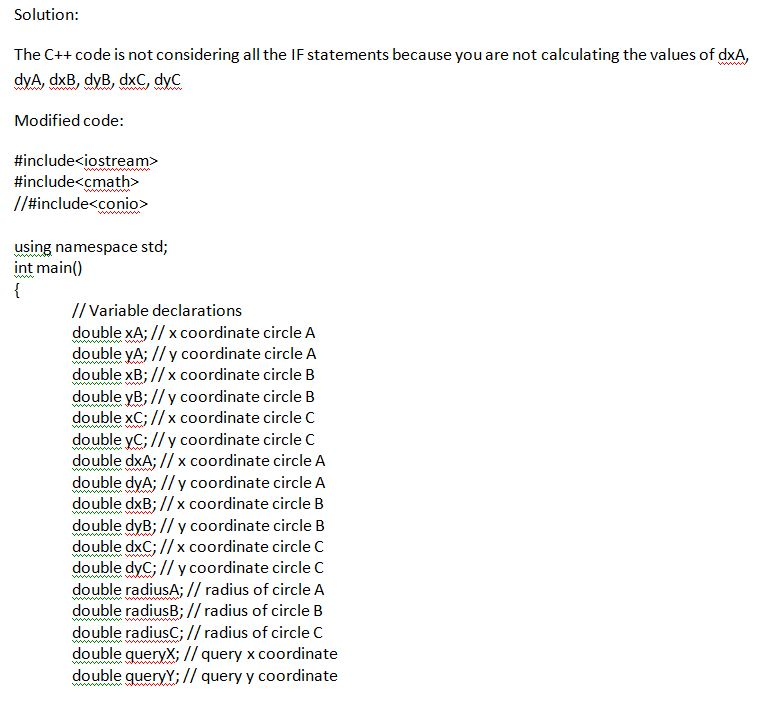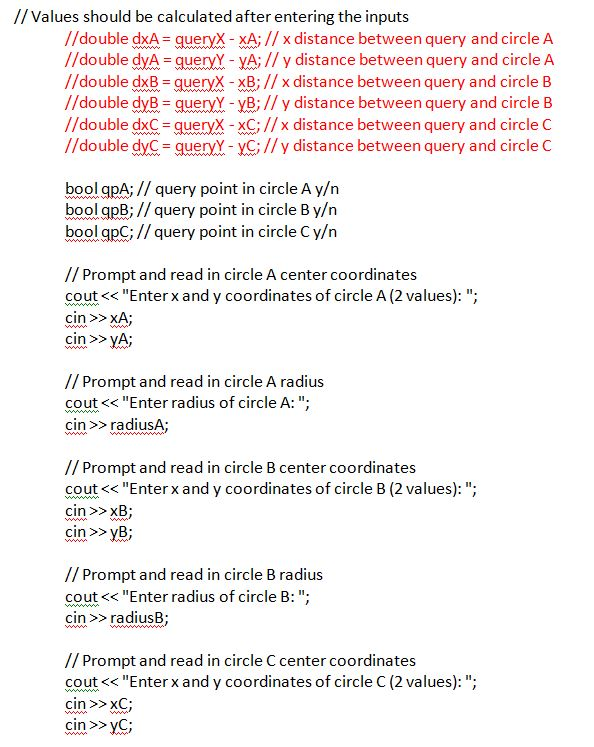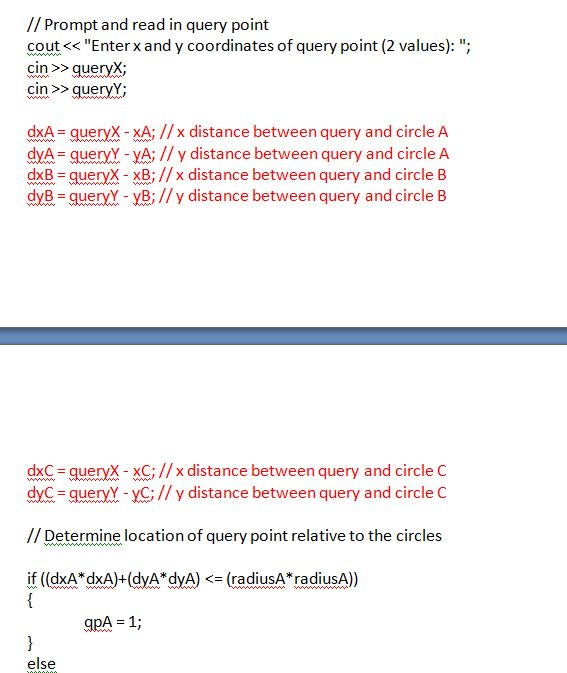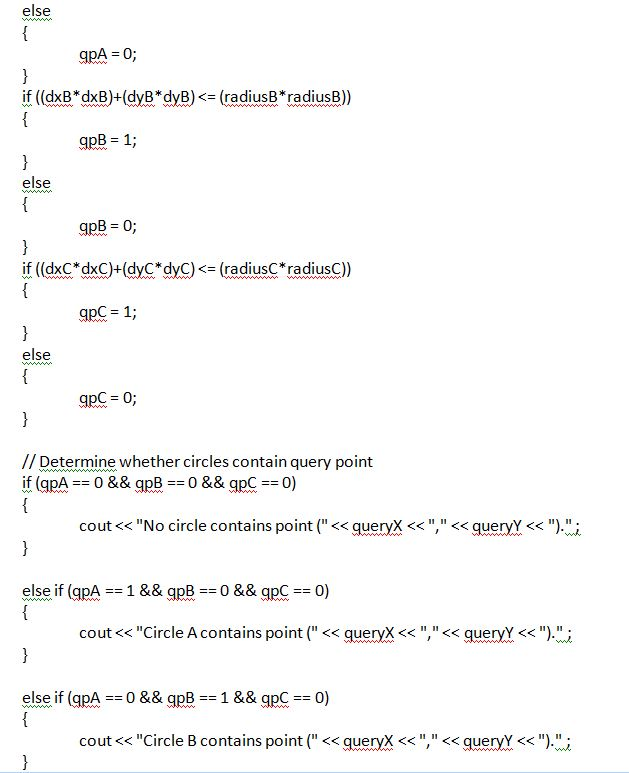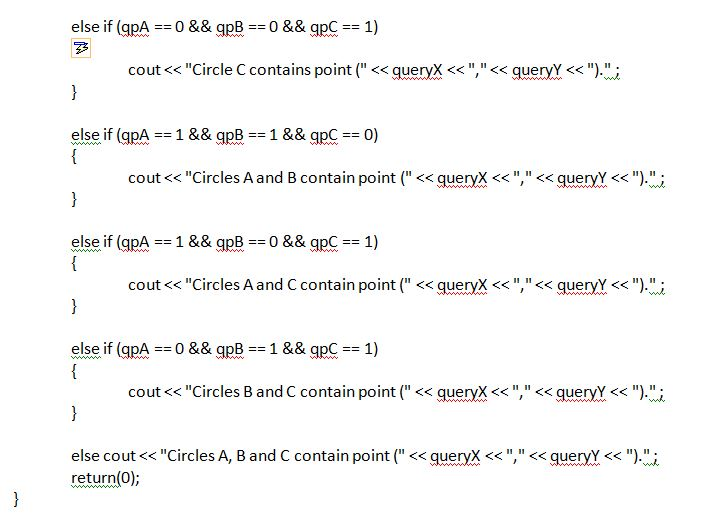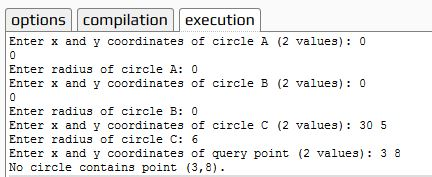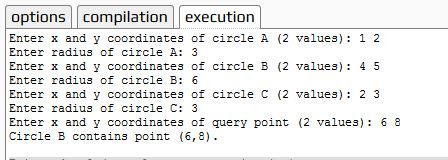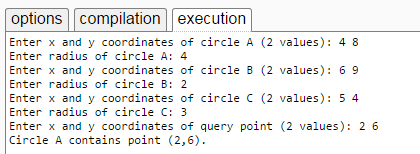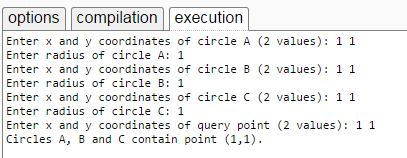Code:

#include<iostream>
#include<cmath>
//#include<conio>

using namespace std;
int main()
{
// Variable declarations
double xA; // x coordinate circle A
double yA; // y coordinate circle A
double xB; // x coordinate circle B
double yB; // y coordinate circle B
double xC; // x coordinate circle C
double yC; // y coordinate circle C
double dxA; // x coordinate circle A
double dyA; // y coordinate circle A
double dxB; // x coordinate circle B
double dyB; // y coordinate circle B
double dxC; // x coordinate circle C
double dyC; // y coordinate circle C
double queryX; // query x coordinate
double queryY; // query y coordinate
//double dxA = queryX – xA; // x distance between query and circle A
//double dyA = queryY – yA; // y distance between query and circle A
//double dxB = queryX – xB; // x distance between query and circle B
//double dyB = queryY – yB; // y distance between query and circle B
//double dxC = queryX – xC; // x distance between query and circle C
//double dyC = queryY – yC; // y distance between query and circle C
bool qpA; // query point in circle A y/n
bool qpB; // query point in circle B y/n
bool qpC; // query point in circle C y/n

// Prompt and read in circle A center coordinates
cout << “Enter x and y coordinates of circle A (2 values): “;
cin >> xA;
cin >> yA;

cout << “Enter radius of circle A: “;

// Prompt and read in circle B center coordinates
cout << “Enter x and y coordinates of circle B (2 values): “;
cin >> xB;
cin >> yB;

cout << “Enter radius of circle B: “;

// Prompt and read in circle C center coordinates
cout << “Enter x and y coordinates of circle C (2 values): “;
cin >> xC;
cin >> yC;

cout << “Enter radius of circle C: “;

// Prompt and read in query point
cout << “Enter x and y coordinates of query point (2 values): “;
cin >> queryX;
cin >> queryY;

dxA = queryX – xA; // x distance between query and circle A
dyA = queryY – yA; // y distance between query and circle A
dxB = queryX – xB; // x distance between query and circle B
dyB = queryY – yB; // y distance between query and circle B
dxC = queryX – xC; // x distance between query and circle C
dyC = queryY – yC; // y distance between query and circle C

// Determine location of query point relative to the circles

{
qpA = 1;
}
else
{
qpA = 0;
}
{
qpB = 1;
}
else
{
qpB = 0;
}
{
qpC = 1;
}
else
{
qpC = 0;
}

// Determine whether circles contain query point
if (qpA == 0 && qpB == 0 && qpC == 0)
{
cout << “No circle contains point (” << queryX << “,” << queryY << “).” ;
}

else if (qpA == 1 && qpB == 0 && qpC == 0)
{
cout << “Circle A contains point (” << queryX << “,” << queryY << “).” ;
}

else if (qpA == 0 && qpB == 1 && qpC == 0)
{
cout << “Circle B contains point (” << queryX << “,” << queryY << “).” ;
}

else if (qpA == 0 && qpB == 0 && qpC == 1)
{
cout << “Circle C contains point (” << queryX << “,” << queryY << “).” ;
}

else if (qpA == 1 && qpB == 1 && qpC == 0)
{
cout << “Circles A and B contain point (” << queryX << “,” << queryY << “).” ;
}

else if (qpA == 1 && qpB == 0 && qpC == 1)
{
cout << “Circles A and C contain point (” << queryX << “,” << queryY << “).” ;
}

else if (qpA == 0 && qpB == 1 && qpC == 1)
{
cout << “Circles B and C contain point (” << queryX << “,” << queryY << “).” ;
}

else cout << “Circles A, B and C contain point (” << queryX << “,” << queryY << “).” ;
return(0);
}Order NOW for a 10% Discount
Pages (550 words)
Approximate price: -

Why Us?Top Quality and Well-Researched Papers

All ourbpapers are written from scratch. In fact, Clients who ask for paraphrasing services are highly discouraged. We have writers ready to craft any paper from scratch and deliver quality ahead of time.Our writers keeps you posted on your papers progress - providing you with paper outline/draft. You are also at liberty to communicate directly with your writer.Free Unlimited Revisions

If you think we missed something, send your order for a free revision. You have 10 days to submit the order for review after you have received the final document. You can do this yourself after logging into your personal account or by contacting our support.Prompt Delivery and 100% Money-Back-Guarantee

All papers are always delivered on time, in many cases quite ahead of time. In case we need more time to master your paper, we may contact you regarding the deadline extension. In case you cannot provide us with more time, a 100% refund is guaranteed.Original & Confidential

We use several writing tools checks to ensure that all documents you receive are free from plagiarism. Our editors carefully review all quotations in the text. We also promise maximum confidentiality in all of our services.Our support agents are available 24 hours a day 7 days a week and committed to providing you with the best customer experience. Get in touch whenever you need any assistance.

Try it now!

## Calculate the price of your order

Total price:
\$0.00

How it works?Fill in the order form and provide all details of your assignment.Proceed with the payment

Choose the payment system that suits you most.Our Services

No need to work on your paper at night. Sleep tight, we will cover your back. We offer all kinds of writing services.## Essay Writing Service

No matter what kind of academic paper you need and how urgent you need it, you are welcome to choose your academic level and the type of your paper at an affordable price. We take care of all your paper needs and give a 24/7 customer care support system.An admission essay is an essay or other written statement by a candidate, often a potential student enrolling in a college, university, or graduate school. You can be rest assurred that through our service we will write the best admission essay for you.Editing Support

Our academic writers and editors make the necessary changes to your paper so that it is polished. We also format your document by correctly quoting the sources and creating reference lists in the formats APA, Harvard, MLA, Chicago / Turabian.Revision Support

If you think your paper could be improved, you can request a review. In this case, your paper will be checked by the writer or assigned to an editor. You can use this option as many times as you see fit. This is free because we want you to be completely satisfied with the service offered.